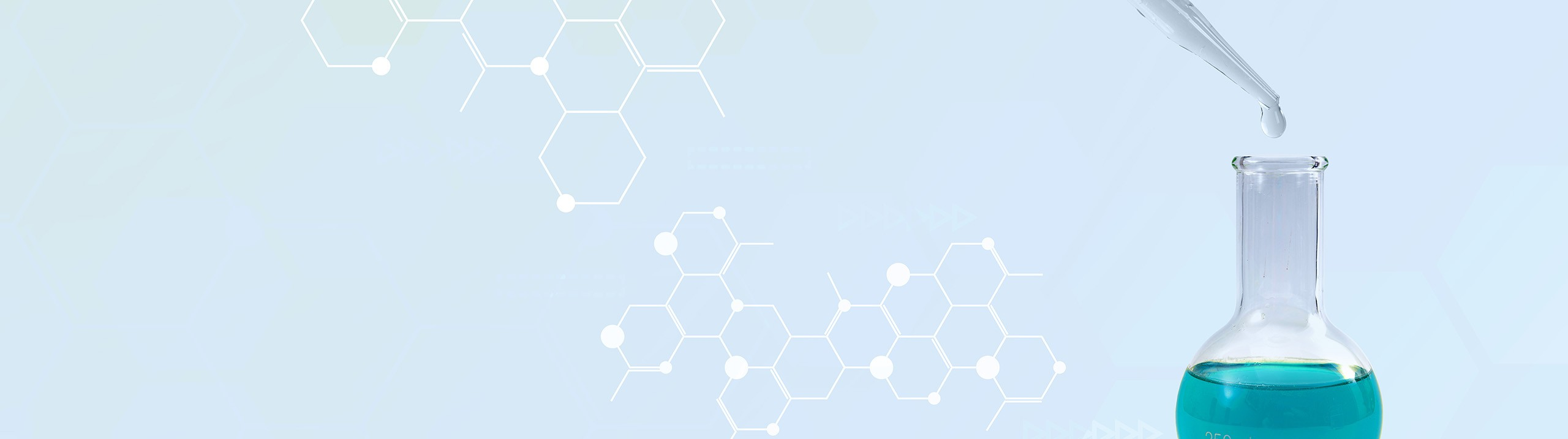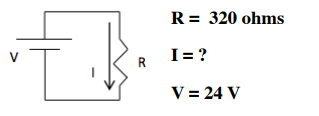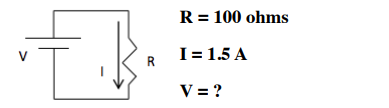## Analytical Chemistry

Learn the toughest concepts covered in your Analytical Chemistry class with step-by-step video tutorials and practice problems.

13. Fundamentals of Electrochemistry

# Basic Concepts

Basic Redox Concepts
1
concept

## Basic Redox3m
Play a video:
So recall that oxidation reduction reactions or redox reactions involves the transferring of electrons from one reactant to another. Now here in our equation we have lithium solid reacting with chlorine gas and in this process we produce lithium ion as well as two chloride ions. Now, remember when it comes to redox reactions, we have oxidation and reduction. To help us remember key concepts. In terms of those two words we use leo the lion goes remember leo means that we're losing electrons and therefore represent oxidation when you're losing negatively charged electrons. The species or element in this case is becoming more positive. That's because it's losing something negative. Now, what exactly about that element is becoming more positive? Well, it's oxidation number is becoming more positive As a result, we're gonna say here that if you are being oxidized then you represent the reducing agent or the reductive. In this case we have lithium solid in its natural state. So its oxidation number is equal to zero. Now, as an eye on its oxidation number is tied to its charge. So here would be plus one. So for lithium it goes from being zero to plus one. So its oxidation number has increased. Therefore it has been oxidized. Therefore it is the reducing agent or the reductive on the other side. When we gain electrons, we're gaining negative electrons. That means the species or the element in this case is becoming more negative as a result of this, the oxidation number decreases here. If you are being reduced, then you represent the oxidizing agent or the oxidant. In this case cl two gas. It's in its natural or elemental state, so its oxidation number is equal to zero. Now, as an ion, its oxidation number is tied to its charge. Each chloride ion is -1, so each chloride ion is -1 in terms of oxidation. Together, they would be minus two, we would say here that we said lithium was being oxidized, so it's losing electrons. It loses in this case, technically would lose two electrons, and chlorine itself would pick up those two electrons because each chlorine needs to gain an electron so each chlorine becomes a chloride ion. As you go deeper and deeper into redox reactions will talk about concepts such as self potential, the use of electrochemical cells as of as well as other concepts related to charge or voltage. Now that you've gotten down the basics of redox reactions, click on the next video and see the different types of variables tied to the transferring of electrons between react ints.
2
concept

## Basic Redox7m
Play a video:
Basic Redox Concepts Calculations
3
example

## Basic Redoxs Calculations 11m
Play a video:
here it states what happens to the current in a circuit? If a three volt battery is removed and replaced by a one volt battery? Now from the previous formulas that we've gone over, we know that current which is I equals your charge E divided by your resistance. R. Remember here that the units for our charge here would be cute and our resistance here would be in homes. So if we think about this, we have current Here. It's 3V Were not given a resistance which means our resistance is being kept the same in both cases. Um so here let's say our resistance is .50 OEMs. So when we worked that out, that would give us a current of six. Remember the units for current are cool items per second And now we've replaced it with one volt. Our resistance can stay the same. So 0.50 homes. And now that's two Cool ems per second. So we can see that our current has gone down and if this current were attached to a light bulb because the current has decreased, that light bulb would become dimmer because we've replaced it um from a three volt battery to a one volt battery. So those are the real world applications of changing the voltage connected to any type of electrical circuit. Now that we've seen this basic question. In terms of currents, let's take a look at example, two in the next question, attempt to do it on your own based on the formulas that I showed on the previous page. If you get stuck, don't worry, just come back and see how I approach that same exact example question.
4
example

## Basic Redoxs Calculations 11m
Play a video:
So here it says if the voltage of a T. E. Series enhanced balance has a 240 volt battery, what is the resistance in the circuit? If the current is 2400.80 amperes. Alright so we're asking to determine resistance. So are we have amperes which is just our current. So that is I. And then we have voltage here which is E. So our equation as before is I equals E. Over R. Rearranging this to isolate our by itself. We see that our equals my voltage divided by my current. So here we'll plug in our voltage of 240V divided by our current of 0.80 amperes. So that gives me 300 gems as my resistance. So that would be our example. Well that would be our answer in terms of this example. So just remember all we're doing here is using the law and just rearranging it to solve for the missing variable which in this case is resistance. Move on to practice question one where we're shown an image of a circuit but again, we're still basing this all off of homes law. So it's just solving for the missing variable based on the information provided
5
Problem

Solve for the missing variable in the following circuit.6
Problem

Solve for the missing variable in the following circuit.7
example

## Basic Redoxs Calculations 23m
Play a video:
here. It says to calculate gibbs free energy under standard conditions for our reaction as well as our self potential. Under standard conditions for redox reaction with N equals four. That has an equilibrium constant of K equals 40.130 at 25 degrees Celsius. Alright, so here remember that N represents our moles of electrons. So we have four moles of electrons. And we're going to say here that the connection between our equilibrium constant K and r delta G of reaction under standard conditions is delta G of reaction equals negative R T L N K. So this involves utilizing the equation that we saw in the past to connect our equilibrium constant. K two delta G. So here R is negative 8.315 here or 8.314472 jewels over k times moles Temperature needs to be in Kelvin. So we're gonna add 73.15-25°C To give us 2 98 0.15 Calvin. And then we're gonna have Ln of K which is 0.130. Here are kelvin's cancel out. So our delta G value comes out to negative 3, 22.3 jewels over moles. So that's how we get our delta G in the beginning. Now we're gonna have to form a connection between delta G and our cell potential. So here the equation that connects them is delta G of our reaction equals negative N times f times E cell. So we're gonna input the value that we just got in terms of delta G, so negative 3, 22.3 jewels over moles Equals moles of electrons transferred which is four moles of electrons Times Faraday's constant, which is 9.647 times 10 to the four columns over moles of electrons. And we don't know what? S all is, that's what we're looking for. So divide out this portion here to isolate our sl so negative four moles of electrons And then 9.64, 7 times 10 to the four columns over moles of electrons on both sides. So when we work that out, we have multiple electrons cancel out. So here, what we're gonna have at the end for S. O. Is really in terms of jewels over coolants. So here this comes out to 8.35 times 10 to the negative four jules over columns. Remember jules over columns. Really just means volts. So this represents our self potential. So remember this question utilized questions um formulas that we've seen in the past in order to form a connection between these three different variables of your equilibrium constant. K Your gibbs free energy of the reaction. Under standard conditions and the cell potential of the reaction. Under standard conditions. Now that we've taken a look at this example, move onto the next one here, we're gonna apply stoke eom a tree to the whole idea of current were given 4.3 amps and some mass of copper. And from that we need to determine the amount of time that has elapsed. So attempt to do this on your own. But if you get stuck, don't worry, come back and see how I approach that same example to question.
8
example

## Basic Redoxs Calculations 23m
Play a video:
copper can be electro plated at the cathode of an electrolysis cell by the half reaction of copper two plus ion plus two electrons produces one mole of copper solid. Here we were asked, how much time would it take for 525 mg of copper to be plated at a current of 4.3 amperes. Alright, so we're looking for time, it doesn't specify if we want to find it in minutes, seconds, years etc. So here we're just gonna figure it out in terms of seconds. Now, remember an amp or an ampere represents the unit for current. And remember an ampere is equal to um charge per over time. So charge or columns over s for seconds. So 4.3 amperes represents 4.3 columns per second. We're gonna first start out with 525 mg of copper. Were they going to convert those milligrams of copper into grams of copper? So one mg of copper is equal to 10 to the negative three g of copper. So milligrams cancel out now we have grams of copper. We're next going to change those grams into moles. So one mole of copper, according to the periodic table weighs 63.546g for copper. And now that I have moles of copper, I can say for my equation that for every one mole of copper solid, we have two moles of electrons involved. So for every one mole of copper, from my balanced equation there are two moles of electrons involved, moles of electrons is really a part of Faraday's constant. So we're gonna say we have one mole of electrons and according to Faraday's constant, that's equal to 9.64, 7 times 10 to the four columns. Finally we have columns which can cancel out with these cool lumps, leaving us with seconds at the end. So we put 4.3 columns on the bottom, one second on top. So now we're gonna have seconds remaining Here, we're gonna get 3.7 times 10 to the two seconds involved. So this is a typical electrochemical question where we're tying charge current two story geometry in some way. Using the balanced equation, we see that the ratio is for every one mole of our solid metal, we have two moles of electrons involved. That comparison there is what allows us to find a way of connecting current to store geometry. Now that we've seen this example. Move on to the final question on this page where we have to determine molar mass of the metal from the question. Now realize what are the units for molar mass. That is key to answering the question correctly. Once you've attempted it, come back and see if you can match your answer with what I get. So good luck guys
9
Problem

A metal forms the salt MCl 3. Electrolysis of the molten salt with a current of 0.700 A for 6.63 h produced 3.00 g of the metal. What is the molar mass of the metal?

10
example

## Basic Redoxs Calculations 33m
Play a video:
So here it states in the following reaction, one mole of zinc solid plus one mole of copper, two sulfate react in order to produce one mole of zinc sulfate plus one mole of copper solid here? It asks, what is the maximum energy produced when 15 g of zinc is completely reacted in a zinc copper electrochemical cell that has an average cell potential of 1.10 volts. Alright, so they're asking us for the maximum amount of energy here. This is equivalent to them asking what's the maximum amount of work that can be done? So what they're asking us to figure out is delta G. And here they give us the mass of zinc and they're giving us self potential. So they're giving us sl realize that the formula that connects delta G to e cell is delta G equals negative N times f times sl here we have to determine the number of electrons transferred. Well, here's ink is zero, but when it's here with sulfate it's plus two because sulfate is minus two. So that's two electrons transferred At the same time. Copper here has to be plus two and here at zero. So we have two electrons involved that are being transferred. So that means N is two. So we have two moles of electrons. We're gonna multiply it by Faraday's constant 9.647 times 10 to the four columns over moles of electrons. And then we're gonna multiply by my voltage. Remember Vault is equal to jules over columns. So that's equal to 1.10 jewels per cool. Um so what happens here is moles of electrons cancel out? Cool um cancel out. So all have jewels of electrons. So that comes out to negative 2.12 times 10 to the five jewels per mole. Now here's the thing, this is the answer when we're dealing with exactly one mole of zinc solid, but in the question, we're not dealing with exactly one mole of zinc solid. What we're dealing with is 15 g of zinc, which is less than one mole. So we're gonna do here is we're gonna take those 15 g, we're gonna convert them into moles. So we're gonna stay here for every one mole of zinc, The Masses, 65.409 g of zinc. So grams of zinc cancel out. Now I have moles of zinc And we're gonna stay here for every one mole of zinc. We found this value here. So it's negative 2.12 times 10 to the five jewels. So this council out with this. So we get at the end is negative 4.87 times 10 to the four jewels. So you can say here that we produce 4.87 times 10 to the four jewels as a result of dealing with just 15 g of zinc. Remember in this type of question, realize what variables are they giving us? And how are they connected together in terms of a formula. Knowing that part allowed us to determine what the energy released when it comes to one mole of zinc, realizing the question that we're dealing with less than a mole of zinc. So what would the new amount of energy be involved Now that you've seen this example? Move on to example, to look to see if you can answer it if you get stuck. Don't worry, come back and see how I approach example, too.
11
example

## Basic Redoxs Calculations 35m
Play a video:
12
example

## Basic Redoxs Calculations 32m
Play a video:
So in this example it says determine the amount of time in minutes needed to produce 1.7 times 10 to the two watts from 1500 jewels of work committed. Alright, so they're giving us watts and we're talking about work, we know that those two variables are connected to power. So power equals work over time. We're gonna say here power using units of watts and a watt is equal to jewels per second. So that's 1.7 times 10 to the two jewels per second. And then we're gonna say here our work is 1500 jewels and we don't know what our time is, that's our X. All we have to do here is isolate X, which will give us seconds. So multiply both sides by X. So 1.7 times 10 to the two jewels over seconds, times X equals 1500 jewels, Divide both sides here by 1.7 times 10 to the two jewels per second Jules Counselor will have seconds. So x equals 8.82 seconds and then you just have to change seconds into minutes. So one minute Is equal to 60 seconds. So that gives me .147 Or .15 minutes. So that's the amount of time it would take. So just remember all the different formulas that utilize in some way. Um questions dealing with energy with current with charge. They are separate from each other but they are connected to each other in one way or another. As we saw an example too. So keep in mind the different formulas that you will have to utilize at some point, whether it's asking to solve for power for current or energy.
Balancing Redox Reactions
13
concept

## Balancing Redox Reactions5m
Play a video:
14
example

## Balancing Redox Reactions Calculations4m
Play a video: